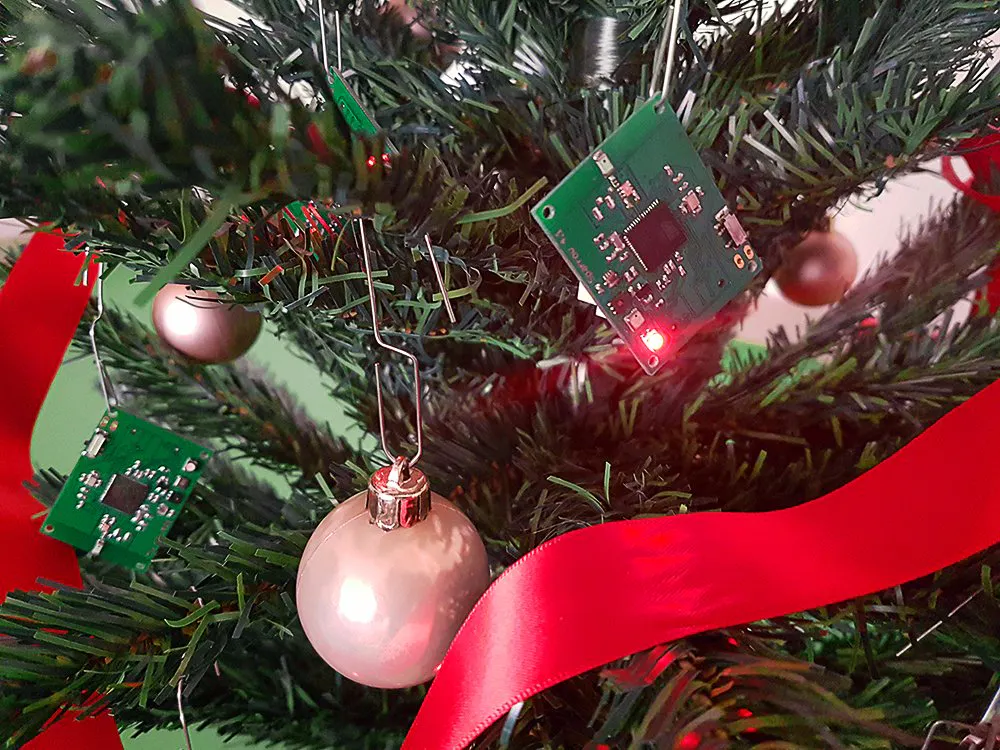# IoT Christmas Tree

Build your own IoT-enabled Christmas tree with the Sparrow Wireless Sensor Nodes!

IntermediateFull instructions provided2 hours372## Things used in this project

### Hardware components

 Sparrow Wireless Sensor Nodes
×5

### Software apps and online servicesArduino IDE

## Code

### The Nodes

Arduino
Program each node with the code below
```#include "SparrowTransfer.h"
#define NODEADDR 8 //this should be unique for each node (range 0-254)
SparrowTransfer ST;
//put your variable definitions here for the data you want to send
//THIS MUST BE EXACTLY THE SAME ON THE OTHER ARDUINO
uint8_t red;
uint8_t green;
uint8_t blue;
};
//give a name to the group of data
// Variables will change:
int ledState = LOW;             // ledState used to set the LED
unsigned long previousMillis = 0;        // will store last time LED was updated
unsigned long currentMillis = 0;
// the follow variables is a long because the time, measured in miliseconds,
// will quickly become a bigger number than can be stored in an int.
long interval = 1000;           // interval at which to blink (milliseconds)
// pins for the LEDs:
const int redPin = 8;
const int greenPin = 11;
const int bluePin = 10;
int red = 0xFF;   //variables to store the current color intensity for each channel
int green = 0xFF; //the LED works on reverse logic, so writing a 0x00 will completely turn on the respective LED
void setup() {
// initialize serial:
Serial.begin(9600);
// make the pins outputs:
pinMode(redPin, OUTPUT);
pinMode(greenPin, OUTPUT);
pinMode(bluePin, OUTPUT);
//start the library, pass in the data details
ST.begin(details(mydata));
}
void ledColor(byte red, byte green, byte blue) //sets the color of the RGB led on the sparrow node
{
analogWrite(redPin, red);
analogWrite(greenPin, green);
analogWrite(bluePin, blue);
}
{
currentMillis = millis();
if(currentMillis - previousMillis > interval) {
// save the last time you blinked the LED
previousMillis = currentMillis;
// if the LED is off turn it on and vice-versa:
if (ledState == LOW)
{
ledState = HIGH;
ledColor(0xFF, 0xFF, 0xFF); //turn the LED off
}
else
{
ledState = LOW;
ledColor(red, green, blue);  // write the current color
}
}
}
void loop() {
if(ST.receiveData()){ //if a new data packet arrived, alter the current color of the LED
{
red = mydata.red;
green = mydata.green;
blue = mydata.blue;
}
}
}
```

### The Coordinator Node

Arduino
```#include "SparrowTransfer.h"
//create object
SparrowTransfer ST;
struct SEND_DATA_STRUCTURE{
//put your variable definitions here for the data you want to send
//THIS MUST BE EXACTLY THE SAME ON THE OTHER ARDUINO
uint8_t red;
uint8_t green;
uint8_t blue;
};
//give a name to the group of data
SEND_DATA_STRUCTURE mydata;
void setup() {
// initialize serial:
Serial.begin(9600);
//start the library, pass in the data details
ST.begin(details(mydata));
}
void loop() {
while (Serial.available() > 0) { //data packets should arrive in csv format, ended by a '\n'
red = Serial.parseInt();     // get the value for the red channel
green = Serial.parseInt();   // get the value for the green channel
blue = Serial.parseInt();    // last value should be the blue channel
// look for the newline. That's the end of a packet
// constrain the values to 0 - 255 and invert because our LED is a common-cathode one
red = 255 - constrain(red, 0, 255);
green = 255 - constrain(green, 0, 255);
blue = 255 - constrain(blue, 0, 255);
mydata.red = red;
mydata.green = green;
mydata.blue = blue;
ST.sendData(); //resend the packet a few times, just to make sure it gets through
delay(100);
ST.sendData();
delay(100);
ST.sendData();
delay(100);
ST.sendData();
delay(100);
ST.sendData();
delay(100);
// print the three numbers in one string as hexadecimal:
Serial.print(red, HEX);
Serial.print(green, HEX);
Serial.println(blue, HEX);
}
}
}
```

## Credits

### Dan Tudose

5 projects • 31 followers